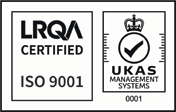## A guide to the weight of common engineering metals, expressed as kgs per cubic metre

 Metal or Alloy Kg /Cu. Mtr Aluminium 2600 Brass 8580 Phosphor Bronze 8850 Cast Iron 7300 Copper 8930 Steel 7850 Titanium 4500 Tungsten 19600 Zinc 7135

## Calculating the weight of a piece of material

### Plate, square and rectangular

To calculate the weight of a plate, square or rectangular piece of material, multiply the width, height and length to get the volume, then multiply by the "Kg /Cu. Mtr" figure for the appropriate material from the above table.

Note: Ensure that all measurements are converted to Meters.

Example:

Find the weight of a steel plate, 2m x 1.5m x 10mm thick.

1) Volume in cubic meters is 2 x 1.5 x 0.01 = 0.03 m3

2) Volume x density , 0.03 x 7850 = 235.5 kg